Question

# An automobile has a mass of 1200 kg. What is its kinetic energy, in kJ, relative...

An automobile has a mass of 1200 kg. What is its kinetic energy, in kJ, relative to the road when traveling at a

velocity of 50 km/h? If the vehicle accelerates to 100 km/h in 15 seconds, what is the change in power required?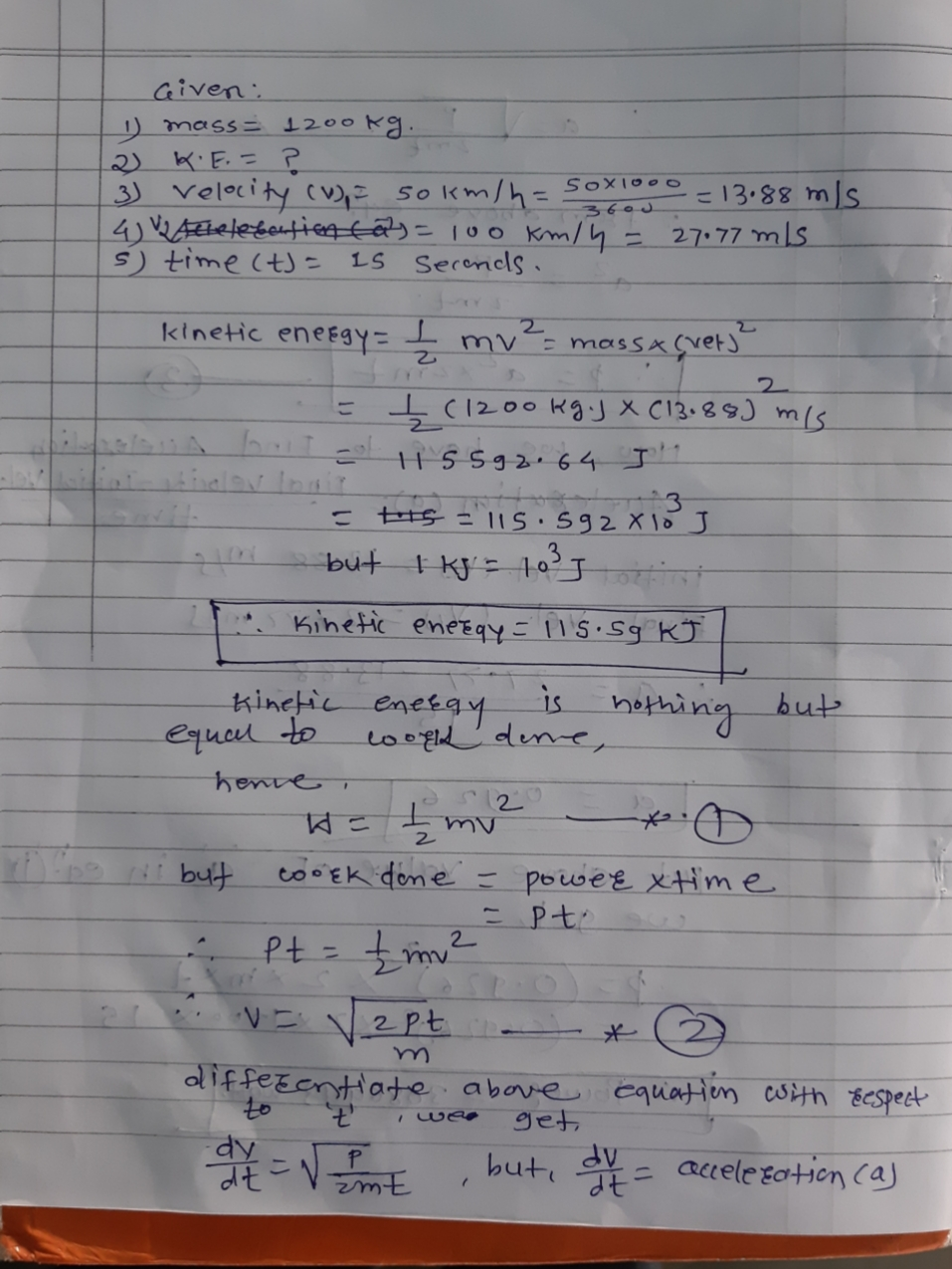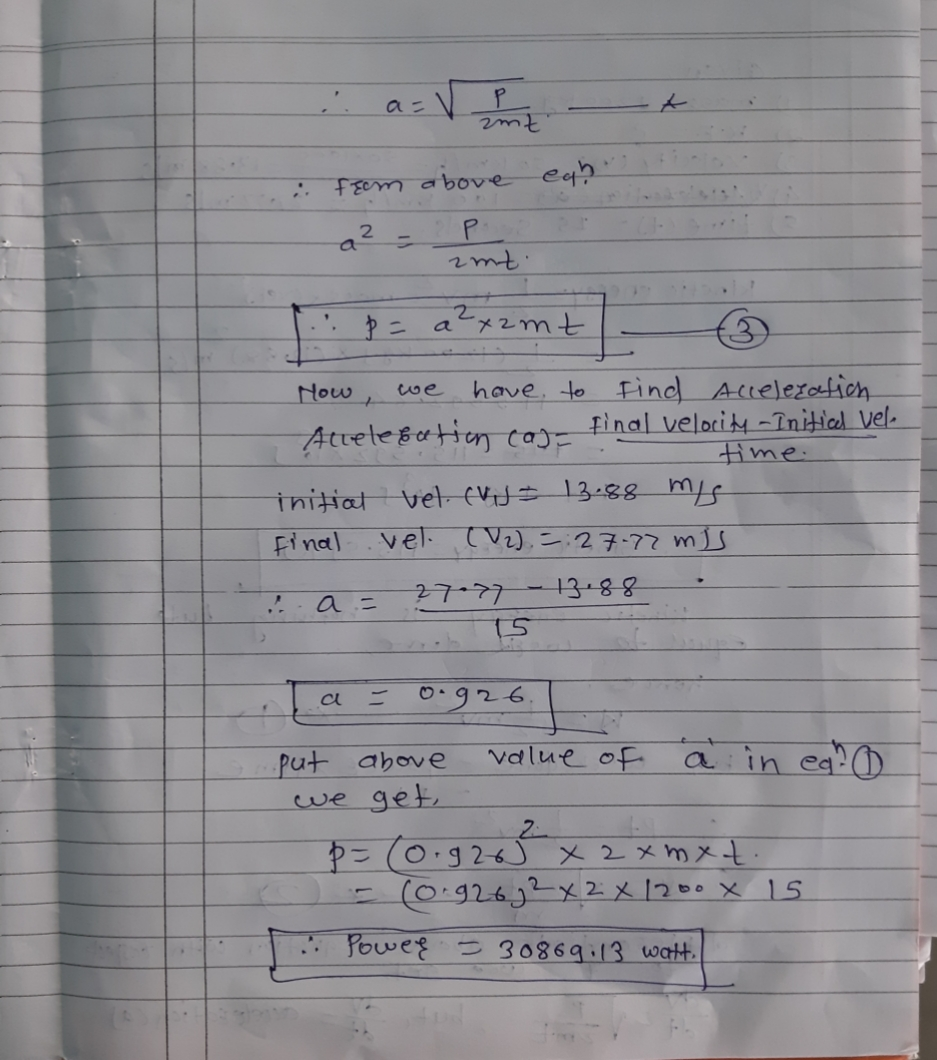#### Earn Coins

Coins can be redeemed for fabulous gifts.

Similar Homework Help Questions
• ### L=200m 1250 Problem 1: A car having a mass of 1200 kg accelerates from 30 to...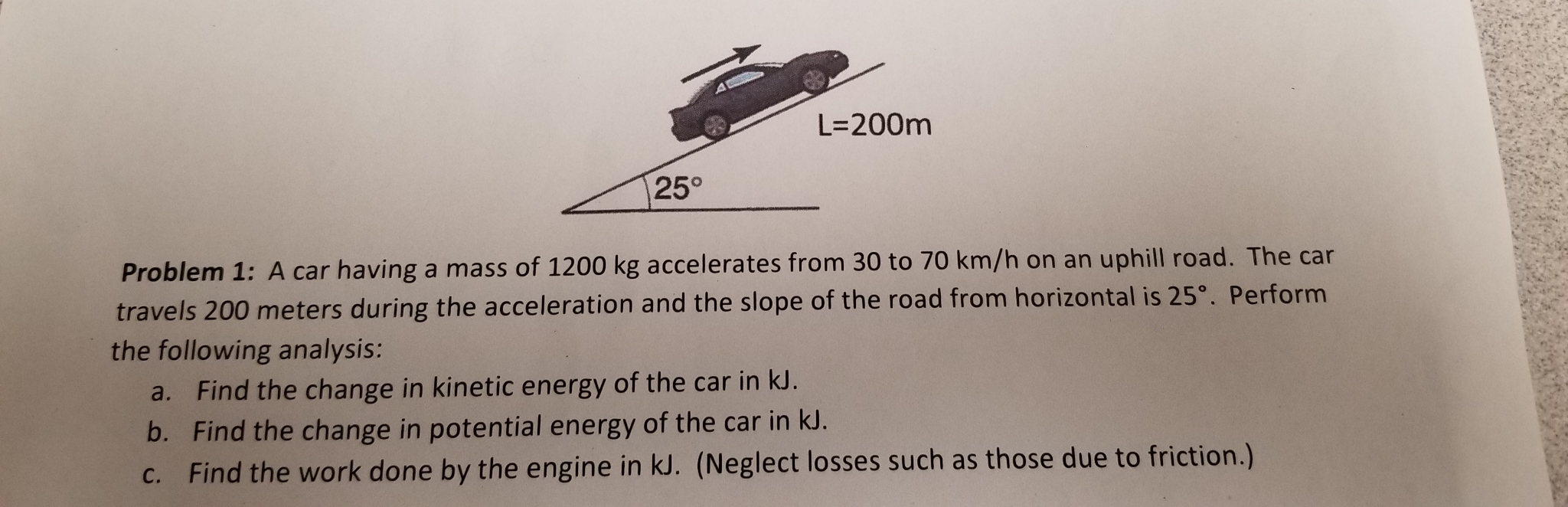L=200m 1250 Problem 1: A car having a mass of 1200 kg accelerates from 30 to 70 km/h on an uphill road. The car travels 200 meters during the acceleration and the slope of the road from horizontal is 25°. Perform the following analysis: a. Find the change in kinetic energy of the car in kl. b. Find the change in potential energy of the car in kJ. c. Find the work done by the engine in kl. (Neglect losses...

• ### An automobile has a total mass of 1100 kg. It accelerates from rest to 69 km/h...

An automobile has a total mass of 1100 kg. It accelerates from rest to 69 km/h in 12 s. Assume each wheel is a uniform 23 kg disk. Find, for the end of the 12 s interval, (a) the rotational kinetic energy of each wheel about its axle, (b) the total kinetic energy of each wheel, and (c) the total kinetic energy of the automobile.

• ### Potential and Kinetic Energy

An automobile having a mass of 900 kg initially moves along a level highway at 100 km/hr relative to the highway. It then climbs a hill whose crest is 50m above thelevel highway and parks at a rest area located there. For the automobile, determine its changes in kinetic and potential energy, each in kJ. For each quantity, kineticenergy and potential energy, specify your choice of datum and reference value at that datum. Let g = 9.81 m/s2

• ### Calculate the kinetic energy (in kJ) of a 3000 kg car that is traveling at a...

Calculate the kinetic energy (in kJ) of a 3000 kg car that is traveling at a speed of 53 km/hr.

• ### A 100-kg disc of radius 50 cm is rotating at 1200 rpm. What is its kinetic...

A 100-kg disc of radius 50 cm is rotating at 1200 rpm. What is its kinetic energy?

• ### The driver of one car has a mass of 1200 kg and is traveling at 10...

The driver of one car has a mass of 1200 kg and is traveling at 10 m/s westward when he collides with another car with a mass of 2000 kg that is traveling 15 m/s northward. The two cars stick together after colliding. Please show all work. a) What is the total momentum before the collision and after the collision? b) What are the speed and direction of the cars after the collision? c) If coefficient of kinetic friction between...

• ### 100 km 03: A crate having mass of 50 kg falls horizontally off the back of...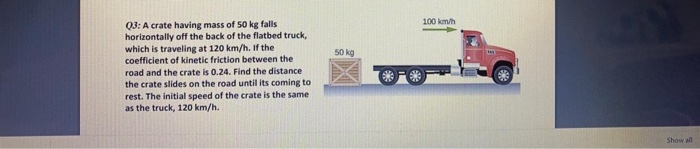100 km 03: A crate having mass of 50 kg falls horizontally off the back of the flatbed truck, which is traveling at 120 km/h. If the coefficient of kinetic friction between the road and the crate is 0.24. Find the distance the crate slides on the road until its coming to rest. The initial speed of the crate is the same as the truck, 120 km/h.

• ### A 2400 kg truck traveling north at 36 km/h turns east and accelerates to 55 km/h....

A 2400 kg truck traveling north at 36 km/h turns east and accelerates to 55 km/h. (a) What is the change in the truck's kinetic energy? (b) What is the magnitude of the change in its momentum?

• ### A 1200 kg frictionless roller coaster starts from rest at a height of 20 m. What is its kinetic...

A 1200 kg frictionless roller coaster starts from rest at a height of 20 m. What is its kinetic energy when it goes over a hill that is 13 m high?in kJ

• ### A vehicle of mass 2000 kg has a constant resistance to motion of 3000 N. The...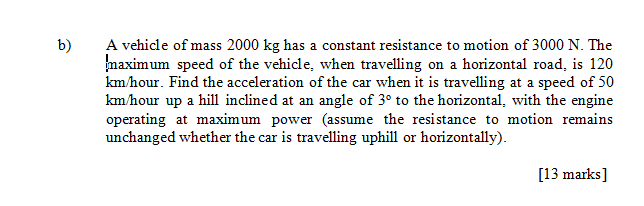A vehicle of mass 2000 kg has a constant resistance to motion of 3000 N. The maximum speed of the vehicle, when travelling on a horizontal road, is 120 km/hour. Find the acceleration of the car when it is travelling at a speed of 50 km/hour up a hill inclined at an angle of 3o to the horizontal, with the engine operating at maximum power (assume the resistance to motion remains unchanged whether the car is travelling uphill or horizonally...